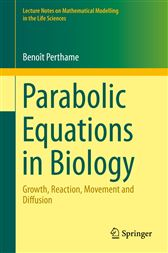# Parabolic Equations in Biology

## Series: Lecture Notes on Mathematical Modelling in the Life SciencesAU\$64.99
###### ISBNs
• 9783319194998
• 9783319195001

This book presents several fundamental questions in mathematical biology such as Turing instability, pattern formation, reaction-diffusion systems, invasion waves and Fokker-Planck equations. These are classical modeling tools for mathematical biology with applications to ecology and population dynamics, the neurosciences, enzymatic reactions, chemotaxis, invasion waves etc. The book presents these aspects from a mathematical perspective, with the aim of identifying those qualitative properties of the models that are relevant for biological applications. To do so, it uncovers the mechanisms at work behind Turing instability, pattern formation and invasion waves. This involves several mathematical tools, such as stability and instability analysis, blow-up in finite time, asymptotic methods and relative entropy properties. Given the content presented, the book is well suited as a textbook for master-level coursework.

###### ISBNs
• 9783319194998
• 9783319195001

#### In The Press

“This book presents a variety of phenomena arising in the analysis of partial differential equations modelling of biological, physical and chemical processes. … This book can well serve as a textbook for a course on master's level. Exercise problems are given in each chapter.” (Jonathan Zinsl, zbMATH 1333.35001, 2016)